## U-, V-, and Dupree statistics

To start, I apologize for this blog’s title but I couldn’t resist referencing to the Owen Wilson classic You, Me, and Dupree – wow! The other gold-plated candidate was U-statistics and You. Please, please, hold your applause.My previous blog post defined statistical functionals as any real-valued function of an unknown CDF,, and explained how plug-in estimators could be constructed by substituting the empirical cumulative distribution function (ECDF)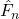for the unknown CDF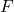. Plug-in estimators of the mean and variance were provided and used to demonstrate plug-in estimators’ potential to be biased.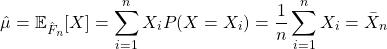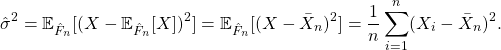Statistical functionals that meet the following two criteria represent a special family of functionals known as expectation functionals:

1)is the expectation of a function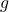with respect to the distribution function; and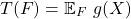2) the functiontakes the form of a symmetric kernel.

Expectation functionals encompass many common parameters and are well-behaved. Plug-in estimators of expectation functionals, named V-statistics after von Mises, can be obtained but may be biased. It is, however, always possible to construct an unbiased estimator of expectation functionals regardless of the underlying distribution function. These estimators are named U-statistics, with the “U” standing for unbiased.

This blog post provides 1) the definitions of symmetric kernels and expectation functionals; 2) an overview of plug-in estimators of expectation functionals or V-statistics; 3) an overview of unbiased estimators for expectation functionals or U-statistics.

## Plug-in estimators of statistical functionals

Consider a sequence of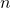independent and identically distributed random variables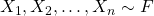. The distribution functionis unknown but belongs to a known set of distribution functions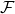. In parametric estimation,may represent a family of distributions specified by a vector of parameters, such as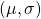in the case of the location-scale family. In nonparametric estimation,is much more broad and is subject to milder restrictions, such as the existence of moments or continuity. For example, we may defineas the family of distributions for which the mean exists or all distributions defined on the real line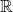.

As mentioned in my previous blog post comparing nonparametric and parametric estimation, a statistical functional is any real-valued function of the cumulative distribution function, denoted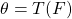. Statistical functionals can be thought of as characteristics of, and include moments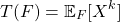and quantiles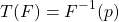as examples.

An infinite population may be considered as completely determined by its distribution function, and any numerical characteristic of an infinite population with distribution functionthat is used in statistics is a [statistical] functional of.

Wassily Hoeffding. “A Class of Statistics with Asymptotically Normal Distribution.” Ann. Math. Statist. 19 (3) 293 – 325, September, 1948.

This blog post aims to provide insight into estimators of statistical functionals based on a sample ofindependent and identically random variables, known as plug-in estimators or empirical functionals.